# Central Science: Chapter 15 Flashcards

Set Details Share
created 2 years ago by Chemistry4Life
2,092 views
updated 2 years ago by Chemistry4Life
College: First year, College: Second year, College: Third year, College: Fourth year
Subjects:
chemistry
Page to share:
Embed this setcancel
COPY
code changes based on your size selection
Size:
X

1

At equilibrium, ________.
A) all chemical reactions have ceased
B) the rates of the forward and reverse reactions are equal
C) the rate constants of the forward and reverse reactions are equal
D) the value of the equilibrium constant is 1
E) the limiting reagent has been consumed

B

2

What role did Karl Bosch play in development of the Haber-Bosch process?
A) He discovered the reaction conditions necessary for formation of ammonia.
B) He originally isolated ammonia from camel dung and found a method for purifying it.
C) Haber was working in his lab with his instructor at the time he worked out the process.
D) He developed the equipment necessary for industrial production of ammonia.
E) He was the German industrialist who financed the research done by Haber.

D

3

Fritz Haber was awarded the ________ Nobel Prize in chemistry for his development of a process for synthesizing ammonia directly from nitrogen and hydrogen.
A) 1954
B) 1918
C) 1933
D) 1900
E) 1912

B

4

Which one of the following is true concerning the Haber process?
A) It is a process used for shifting equilibrium positions to the right for more economical chemical synthesis of a variety of substances.
B) It is a process used for the synthesis of ammonia.
C) It is another way of stating Le Châtelier's principle.
D) It is an industrial synthesis of sodium chloride that was discovered by Karl Haber.
E) It is a process for the synthesis of elemental chlorine.

B

5

Which one of the following will change the value of an equilibrium constant?
A) changing temperature
B) adding other substances that do not react with any of the species involved in the equilibrium
C) varying the initial concentrations of reactants
D) varying the initial concentrations of products
E) changing the volume of the reaction vessel

A

6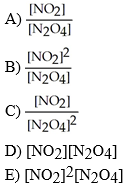Which of the following expressions is the correct equilibrium-constant expression for the equilibrium between dinitrogen tetroxide and nitrogen dioxide?

N2O4 (g) ⇌ 2NO2 (g)

B

7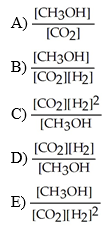Which of the following expressions is the correct equilibrium-constant expression for the following reaction?

CO2 (g) + 2H2 (g) ⇌ CH3OH (g)

E

8

The equilibrium-constant expression depends on the ________ of the reaction.
A) stoichiometry
B) mechanism
C) stoichiometry and mechanism
D) the quantities of reactants and products initially present
E) temperature

A

9

The equilibrium constant for reaction 1 is K. The equilibrium constant for reaction 2 is ________.

(1) SO2 (g) + (1/2) O2 (g) ⇌ SO3 (g)
(2) 2SO3 (g) ⇌ 2SO2 (g) + O2 (g)

A) K2
B) 2K
C) 1/2K
D) 1/K2
E) -K2

D

10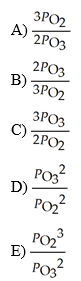The equilibrium expression for Kp for the reaction below is ________.

2O3 (g) ⇌ 3O2 (g)

E

11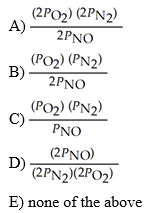The equilibrium expression for Kp for the reaction below is ________.

N2 (g) + O2 (g) ⇌ 2NO (g)

E

12

The Keq for the equilibrium below is 7.52 × 10-2 at 480.0 °C.

2Cl2 (g) + 2H2O (g) ⇌ 4HCl (g) + O2 (g)

What is the value of Keq at this temperature for the following reaction?

2HCl (g) + 1/2 O2 (g) ⇌ Cl2 (g) + H2O (g)
A) 13.3
B) 3.65
C) -0.0376
D) 5.66 × 10-3
E) 0.274

B

13

The Keq for the equilibrium below is 5.4 × 1013 at 480.0 °C.

2NO (g) + O2 (g) ⇌ 2NO2 (g)

What is the value of Keq at this temperature for the following reaction?

2NO2 (g) ⇌ 2NO (g) + O2 (g)
A) 5.4 × 10-13
B) 1.9 × 10-14
C) 5.4 × 1013
D) 5.66 × 10-3
E) none of the above

B

14

The Keq for the equilibrium below is 50.

H2 (g) + I2 (g) ⇌ 2HI (g)

What is the value of Keq for the following reaction?

2HI (g) ⇌ H2 (g) + I2 (g)

A) 100
B) 0.50
C) 0.020
D) 2500
E) -50

C

15

The Keq for the equilibrium below is 50.

H2 (g) + I2 (g) ⇌ 2HI (g)

What is the value of Keq for the following reaction?

1/2 H2 (g) + 1/2 I2 (g) ⇌ HI (g)

A) 25
B) 2500
C) 7.07
D) 100
E) -50

C

16

Given the following reaction at equilibrium, if Kc = 1.90 × 1019 at 25.0 °C, Kp = ________.

H2 (g) + Br2 (g) ⇌ 2 HBr (g)

A) 5.26 × 10-20
B) 1.56 × 104
C) 6.44 × 105
D) 1.90 × 1019
E) none of the above

D

17

Which of the following expressions is the correct equilibrium-constant expression for the reaction below?

2SO2 (g) + O2 (g) ⇌ 2SO3 (g)

A) [SO3] / [SO2][O2]
B) [SO2] / [SO3]
C) [SO3]2 / [SO2]2[O2]
D) [SO3]2 / [SO2]2[O2]2
E) [SO3] / [SO2][O2]2

C

18

Which of the following expressions is the correct equilibrium-constant expression for the reaction below?

(NH4)2Se (s) ⇌ 2NH3 (g) + H2Se (g)

A) [NH3][H2Se] / [(NH4)2Se]
B) [(NH4)2Se] / [NH3]2[H2Se]
C) 1 / [(NH4)2Se]
D) [NH3]2[H2Se]
E) [NH3]2[H2Se] / [(NH4)2Se]

D

19

Which of the following expressions is the correct equilibrium-constant expression for the reaction below?

CO2 (s) + H2O (l) ⇌ H+ (aq) + HCO3- (aq)

A) [H+][HCO3-] / [CO2]
B) [CO2] / [H+][HCO3-]
C) [H+][HCO3-] / [CO2][H2O]
D) [CO2][H2O] / [H+][HCO3-]
E) [H+][HCO3-]

E

20

Which of the following expressions is the correct equilibrium-constant expression for the reaction below?

HF (aq) + H2O (l) ⇌ H3O+ (aq) + F- (aq)

A) [HF][H2O] / [H3O+][F-]
B) 1 / [HF]
C) [H3O+][F-] / [HF][H2O]
D) [H3O+][F-] / [HF]
E) [F-] / [HF]

D

21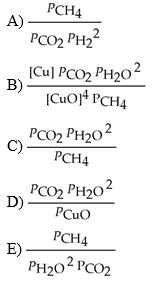The expression for for the reaction below is ________.

4CuO (s) + CH4 (g) ⇌ CO2 (g) + 4Cu (s) + 2H2O (g)

C

22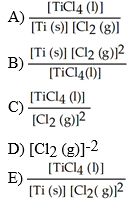The equilibrium-constant expression for the reaction

Ti (s) + 2Cl2 (g) ⇌ TiCl4 (l)

D

23

The equilibrium constant for the gas phase reaction

N2 (g) + 3H2 (g) ⇌ 2NH3 (g)

is Keq = 4.34 × 10-3 at 300 °C. At equilibrium, ________.
A) products predominate
B) reactants predominate
C) roughly equal amounts of products and reactants are present
D) only products are present
E) only reactants are present

B

24

The equilibrium constant for the gas phase reaction

2SO2 (g) + O2 (g) ⇌ 2SO3 (g)

is Keq = 2.80 × 102 at 999 K. At equilibrium, ________.
A) products predominate
B) reactants predominate
C) roughly equal amounts of products and reactants are present
D) only products are present
E) only reactants are present

A

25

The equilibrium constant for the gas phase reaction

2NH3 (g) ⇌ N2 (g) + 3H2 (g)

is Keq = 230 at 300 °C. At equilibrium, ________.
A) products predominate
B) reactants predominate
C) roughly equal amounts of products and reactants are present
D) only products are present
E) only reactants are present

A

26

The equilibrium constant for the gas phase reaction

H2 (g) + I2 (g) ⇌ 2HI (g)

is Keq = 50 at 25 °C. At equilibrium, ________.
A) products predominate
B) reactants predominate
C) roughly equal amounts of products and reactants are present
D) only products are present
E) only reactants are present

A

27

The equilibrium constant for the gas phase reaction

2SO3 (g) ⇌ 2SO2 (g) + O2 (g)

is Keq = 3.6 × 10-3 at 999 K. At equilibrium, ________.
A) products predominate
B) reactants predominate
C) roughly equal amounts of products and reactants are present
D) only products are present
E) only reactants are present

B

28

At 400 K, the equilibrium constant for the reaction

Br2 (g) + Cl2 (g) ⇌ 2BrCl (g)

is Kp = 7.0. A closed vessel at 400 K is charged with 1.00 atm of Br2 (g), 1.00 atm of Cl2 (g), and 2.00 atm of BrCl (g). Use Q to determine which of the statements below is true.
A) The equilibrium partial pressures of Br2, Cl2, and BrCl will be the same as the initial values.
B) The equilibrium partial pressure of BrCl (g) will be 4.00 atm.
C) The equilibrium partial pressure of Br2 will be greater than 1.00 atm.
D) At equilibrium, the total pressure in the vessel will be less than the initial total pressure.
E) The reaction will go to completion since there are equal amounts of Br2 and Cl2.

B

29

Which of the following statements is true?
A) Q does not change with temperature.
B) Keq does not change with temperature, whereas Q is temperature dependent.
C) K does not depend on the concentrations or partial pressures of reaction components.
D) Q does not depend on the concentrations or partial pressures of reaction components.
E) Q is the same as Keq when a reaction is at equilibrium

E

30

How is the reaction quotient used to determine whether a system is at equilibrium?
A) The reaction quotient must be satisfied for equilibrium to be achieved.
B) At equilibrium, the reaction quotient is undefined.
C) The reaction is at equilibrium when Q < Keq.
D) The reaction is at equilibrium when Q > Keq.
E) The reaction is at equilibrium when Q = Keq.

E

31

Which reaction will shift to the left in response to a decrease in volume?
A) 2HI (g) ⇌ H2 (g) + I2 (g)
B) H2 (g) + Cl2 (g) ⇌ 2 HCl (g)
C) N2 (g) + 3H2 (g) ⇌ 2 NH3 (g)
D) 2 SO3 (g) ⇌ 2 SO2 (g) + O2 (g)
E) 4 Fe (s) + 3 O2 (g) ⇌ 2 Fe2O3 (s)

D

32

Which reaction will shift to the right in response to a decrease in volume?
A) N2 (g) + 3H2 (g) ⇌ 2NH3 (g)
B) H2 (g) + Cl2 (g) ⇌ 2 HCl (g)
C) 2 SO3 (g) ⇌ 2 SO2 (g) + O2 (g)
D) 2HI (g) ⇌ H2 (g) + I2 (g)
E) 2 Fe2O3 (s) ⇌ 4 Fe (s) + 3O2 (g)

A

33

Based on Le Châtelier's principle, increasing pressure at constant temperature in the following reaction will not change the concentrations of reactants and products.
A) N2O4 (g) ⇌ 2NO2 (g)
B) N2 (g) + O2 (g) ⇌ 2NO (g)
C) N2 (g) + 2O2 (g) ⇌ 2NO2 (g)
D) N2 (g) + 3H2 (g) ⇌ 2NH3 (g)
E) 2N2 (g) + O2 (g) ⇌ 2N2O (g)

B

34

In which of the following reactions would increasing pressure at constant temperature change the concentrations of reactants and products, based on Le Châteliers principle?
A) N2 (g) + 3H2 (g) ⇌ 2NH3 (g)
B) N2O4 (g) ⇌ 2NO2 (g)
C) N2 (g) + 2O2 (g) ⇌ 2NO2 (g)
D) 2N2 (g) + O2 (g) ⇌ 2 O (g)
E) all of the above

E

35

Consider the following reaction at equilibrium:

2NH3 (g) ⇌ N2 (g) + 3H2 (g)

Le Châtelier's principle predicts that the moles of in the reaction container will increase with ________.
A) some removal of from the reaction vessel (V and T constant)
B) a decrease in the total pressure (T constant)
C) addition of some to the reaction vessel (V and T constant)
D) a decrease in the total volume of the reaction vessel (T constant)
E) an increase in total pressure by the addition of helium gas (V and T constant)

B

36

Consider the following reaction at equilibrium:

2CO2 (g) ⇌ 2CO (g) + O2 (g) ΔH° = -514 kJ

Le Châtelier's principle predicts that a decrease in temperature will ________.
A) decrease the partial pressure of O2 (g)
B) increase the partial pressure of CO2 (g)
C) decrease the value of the equilibrium constant
D) increase the value of the equilibrium constant
E) decrease the partial pressure of CO

D

37

Consider the following reaction at equilibrium.

2CO2 (g) ⇌ 2CO (g) + O2 (g) ΔH° = -514 kJ

Le Châtelier's principle predicts that the equilibrium partial pressure of CO (g) can be maximized by carrying out the reaction ________.
A) at high temperature and high pressure
B) at high temperature and low pressure
C) at low temperature and low pressure
D) at low temperature and high pressure
E) in the presence of solid carbon

C

38

The effect of a catalyst on an equilibrium is to ________.
A) increase the rate of the forward reaction only
B) increase the equilibrium constant so that products are favored
C) slow the reverse reaction only
D) increase the rate at which equilibrium is achieved without changing the composition of the equilibrium mixture
E) shift the equilibrium to the right

D

39

The value of Keq for the equilibrium

H2 (g) + I2 (g) ⇌ 2HI (g)

is 794 at 25 °C. What is the value of Keq for the equilibrium below?

1/2 H2 (g) + 1/2 I2 (g) ⇌ HI (g)

A) 397
B) 0.035
C) 28
D) 1588
E) 0.0013

C

40

The value of Keq for the equilibrium

H2 (g) + I2 (g) ⇌ 2HI (g)

is 794 at 25 °C. At this temperature, what is the value of Keq for the equilibrium below?

HI (g) ⇌ 1/2 H2 (g) + 1/2 I2 (g)

A) 1588
B) 28
C) 397
D) 0.035
E) 0.0013

D

41

The value of Keq for the equilibrium

H2 (g) + I2 (g) ⇌ 2HI (g)

is 54.0 at 427 °C. What is the value of Keq for the equilibrium below?

HI (g) ⇌ 1/2 H2 (g) + 1/2 I2(g)

A) 27
B) 7.35
C) 0.136
D) 2.92 × 103
E) 3.43 × 10-4

C

42

The value of Keq for the equilibrium

CO2 (g) + 2H2 (g) ⇌ CH3OH (g)

is 14.5 at 483 °C. What is the value of Keq for the equilibrium below?

1/2 CO2 + H2 (g) ⇌ 1/2 CH3OH (g)

A) 7.30
B) 7.35
C) 0.136
D) 3.81
E) 6.90 × 10-2

D

43

The value of Keq for the equilibrium

N2 (g) + O2 (g) ⇌ 2 NO (g)

is 4.2 × 10-31 at 27 °C. What is the value of Keq for the equilibrium below?

4 NO (g) ⇌ 2 N2 (g) + 2 O2 (g)

A) 5.7 × 1060
B) 8.4 × 10-31
C) 4.2 × 1031
D) 8.4 × 1031
E) none of the above

A

44

Consider the following chemical reaction:

CO (g) + 2H2(g) ⇌ CH3OH(g)

At equilibrium in a particular experiment, the concentrations of CO and H2 were 0.15M and 0.36M, respectively. What is the equilibrium concentration of CH3OH? The value of Keq for this reaction is 14.5 at the temperature of the experiment.
A) 14.5
B) 7.61 × 10-3
C) 2.82 × 10-1
D) 3.72 × 10-3
E) 1.34 × 10-3

C

45

A reaction vessel is charged with hydrogen iodide, which partially decomposes to molecular hydrogen and iodine:

2HI (g) ⇌ H2(g) + I2(g)

When the system comes to equilibrium at 425 °C, PHI = 0.708 atm, and PH2=PI2=0.0960atm. The value of Kp at this temperature is ________.
A) 6.80 × 10-2
B) 1.30 × 10-2
C) 54.3
D) 1.84 × 10-2
E) Kp cannot be calculated for this gas reaction when the volume of the reaction vessel is not given.

D

46

Acetic acid is a weak acid that dissociates into the acetate ion and a proton in aqueous solution:

HC2H3O2 (aq) ⇌ C2H3O2- (aq) + H+ (aq)

At equilibrium at 25 °C a 0.100 M solution of acetic acid has the following concentrations: |HC2H302| = 0.0990M, |C2H302-| = 1.33 X 10-3M, and |H+| = 1.33 X 10-3M. The equilibrium constant, Keq, for the ionization of acetic acid at is ________.
A) 5.71 × 104
B) 0.100
C) 1.75 × 10-7
D) 1.79 × 10-5
E) 5.71 × 106

D

47

Dinitrogentetraoxide partially decomposes into nitrogen dioxide. A 1.00-L flask is charged with 0.0400 mol of N2O4. At equilibrium at 373 K, 0.0055 mol of N2O4 remains. Keq for this reaction is ________.
A) 2.2 × 10-4
B) 13
C) 0.22
D) 0.87
E) 0.022

D

48

In an experiment, 0.42 mol of CO and 0.42 mol of H2 were placed in a 1.00-L reaction vessel to yield CH3OH. At equilibrium, there were 0.29 mol of CO remaining. Keq at the temperature of the experiment is ________.
A) 2.80
B) 17.5
C) 0.357
D) 14.5
E) none of the above

B

49

A sealed 1.0 L flask is charged with 0.500 mol of I2 and 0.500 mol of Br2. An equilibrium reaction ensues:

I2 (g) + Br2 (g) ⇌ 2IBr (g)

When the container contents achieve equilibrium, the flask contains 0.84 mol of IBr. The value of Keq is ________.
A) 11
B) 4.0
C) 110
D) 6.1
E) 2.8

C

50

The equilibrium constant (Kp) for the interconversion of PCl5 and PCl3 is 0.0121. A vessel is charged with PCl5 giving an initial pressure of 0.123 atm and yields PCl3 and Cl2. At equilibrium, the partial pressure of PCl3 is ________ atm.
A) 0.0782
B) 0.0330
C) 0.0908
D) 0.0455
E) 0.123

B

51

At 200 °C, the equilibrium constant (Kp) for the conversion of NO to oxygen and nitrogen gas is 2.40 × 103. A closed vessel is charged with 36.1 atm of NO. At equilibrium, the partial pressure of O2 is ________ atm.
A) 294
B) 6.00
C) 35.7
D) 1.50 × 10-2
E) 17.9

E

52

Which of the following expressions is the correct equilibrium-constant expression for the equilibrium between dinitrogen tetroxide and nitrogen dioxide?

5N2O4(g) ⇌ 10NO2 (g)

A) [NO2]10/[N2O4]5
B) [N2O4]10/[NO2]5
C) [NO2]5/[N2O4]10
D) [NO2]5/[N2O4]5
E) [N2O4]5/[NO2]5

A

53

Given the following reaction at equilibrium, if Kc = 5.84 x 105 at 230.0 °C, Kp = ________.

2NO (g) + O2 (g) ⇌ 2NO2(g)

A) 3.67 × 10-2
B) 1.41 × 104
C) 6.44 × 105
D) 2.40 × 106
E) 2.41 × 107

B

54

Given the following reaction at equilibrium at 450.0 °C:

CaCO3 (s) ⇌ CaO (s) + CO2 (g)

If pCO2 = 0.0155 atm, Kc = ________.
A) 155
B) 0.0821
C) 0.920
D) 2.61 × 10-4
E) 9.20

D

55

Given the following reaction at equilibrium, if Kp = 1.10 at 250.0 °C, Kc = ________.

PCl5 (g) ⇌ PCl3 (g) + Cl2 (g)

A) 3.90 × 10-6
B) 2.56 × 10-2
C) 1.10
D) 42.9
E) 47.2

B

56

Given the following reaction at equilibrium at 300.0 K:

NH4HS (s) ⇌ NH3 (g) + H2S (g)

If pNH3 = pH2S = 0.105 atm, Kp = ________.
A) .0110
B) 4.99 × 10-4
C) .105
D) .0821
E) 5.66 × 10-3

A

57

The value of Keq for the following reaction is 0.25:

S (g) + N (g) ⇌ SO3 (g) + NO (g)

The value of Keq at the same temperature for the reaction below is ________.

3SO2 (g) + 3NO2 (g) ⇌ 3SO2 (g) + 3NO (g)

A) 1.6 × 10-2
B) 7.5 × 10-1
C) 8.3 × 10-2
D) 6.4 × 101
E) 0.25

A

58

The Keq for the equilibrium below is 7.52 × 10-2 at 480.0 °C.

2Cl2 (g) + 2H2O (g) ⇌ 4HCl (g) + O2 (g)

What is the value of Keq at this temperature for the following reaction?

8HCl (g) + 2O2 (g) ⇌ 4Cl2 (g) + 4H2O (g)

A) 1.77 × 102
B) 5.66 × 10-3
C) 1.50 × 10-1
D) -7.52 × 10-2
E) 7.52 × 10-2

A

59

The Keq for the equilibrium below is 5.4 × 1013 at 480.0 °C.

2NO (g) + O2 (g) ⇌ 2NO2 (g)

What is the value of Keq at this temperature for the following reaction?

4NO (g) + 2O2 (g) ⇌ 4NO2 (g)

A) 2.9 × 1027
B) 8.5 × 1054
C) 3.4 × 10-28
D) -1.1 × 1014
E) 5.4 × 1013

A

60

The Keq for the equilibrium below is 0.112 at 700.0 °C.

SO2 (g) + 1/2 O2 (g) ⇌ SO3 (g)
What is the value of Keq at this temperature for the following reaction?

2SO2 (g) + O2 (g) ⇌ 2SO3 (g)

A) 1.25 × 10-2
B) 2.24 × 10-1
C) 7.97 × 101
D) 4.46
E) 0.112

A

61

The value of Keq for the following reaction is 0.26:

A (g) + B (g) ⇌ C (g) + D (g)

The value of Keq at the same temperature for the reaction below is ________.

2A (g) + 2B (g) ⇌ 2C (g) + 2D (g)

A) 0.068
B) 0.52
C) 1.2
D) 0.065
E) 0.26

A

62

The value of Keq for the following reaction is 0.16:

A (g) + B (g) ⇌ C (g) + D (g)

The value of Keq at the same temperature for the reaction below is ________.

3C (g) + 3D (g) ⇌ 3A (g) + 3B (g)

A) 2.4 × 102
B) 2.1
C) 4.1 × 10-3
D) 5.3 × 10-2
E) 6.3

A

63

The value of Keq for the following reaction is 0.50:

A (g) + 2B (g) ⇌ C (g) + 4D (g)

The value of Keq at the same temperature for the reaction below is ________.

1/2A (g) + B (g) ⇌ 1/2C (g) + 2D (g)

A) 7.1 × 10-1
B) 2.5 × 10-1
C) 0.25
D) 1.0
E) 0.50

A

64

The Keq for the equilibrium below is 7.16 × 10-2 at 440.0 °C.

2Cl2 (g) + 2H2O (g) ⇌ 4HCl (g) + O2 (g)

What is the value of Keq at this temperature for the following reaction?
Cl2 (g) + H2O (g) ⇌ 2HCl (g) + 1/2 O2 (g)
A) 0.0716
B) 5.13 × 10-3
C) 0.268
D) 0.0376
E) 0.150

C

65

At 1000.0 K, the equilibrium constant for the reaction

2NO (g) + Br2 (g) ⇌ 2NOBr (g)

is Kp = 0.016. Calculate Kp for the reverse reaction,

2NOBr (g) ⇌ 2NO (g) + Br2 (g).
A) 0.016
B) 1.6 × 10-4
C) 63
D) 0.99
E) 1.1

C

66

The expression of Keq for the following reaction will not include ________.

A(g) + B(g) ⇌ C(l) + D(g)

A) [C]
B) [A]
C) [B]
D) [D]
E) none of the above

A

67

Phosphorous trichloride and phosphorous pentachloride equilibrate in the presence of molecular chlorine according to the reaction:

PCl3(g) + Cl2(g) → PCl5(g)

An equilibrium mixture at 450 K contains
PPCl3= 0.224 atm,
PCl2= 0.284 atm, and
PPCl5= 4.24 atm. What is the value of Kp at this temperature?
A) 66.7
B) 1.50 ×10-2
C) 2.70 × 10-1
D) 3.74
E) 8.36

A

68

Hydrogen and iodine gas react to produce hydrogen iodide gas. What is the value of Keq for this reaction if at equilibrium the concentrations of H2, I2, and HI were 0.25M, 0.035M, and 0.55M, respectively?
A) 23
B) 34
C) 63
D) 0.0090
E) 5.1

B

69

Dinitrogen tetroxide partially decomposes according to the following equilibrium:

N2O4(g) → 2NO2(g)

A 1.000-L flask is charged with 9.20 × 10-3 mol of N2O4. At equilibrium, 5.98 × 10-3 mol N2O4 of remains. Keq for this reaction is ________.
A) 0.183
B) 0.197
C) 0.212
D) 6.94 ×10-3
E) 2.96 × 10-5

D

70

Carbon monoxide and chlorine gas react to produce COCl2 gas. The Kp for the reaction is 1.49 × 108 at 100.0 °C: In an equilibrium mixture of the three gases, PCO = PCl2 = 7.70 × 10-4 atm. The partial pressure of the product, phosgene (COCl2 ), is ________ atm.
A) 2.51 × 1014
B) 3.98 × 10-15
C) 1.15 × 105
D) 8.83 × 101
E) 1.92 × 1011

D

71

At 900.0 K, the equilibrium constant (Kp) for the following reaction is 0.345.

2SO2 + O2(g) → 2SO3(g)

At equilibrium, the partial pressure of SO2 is 36.9 atm and that of O2 is 16.8 atm. The partial pressure of SO3 is ________ atm.
A) 88.8
B) 3.89 ×10-3
C) 214
D) 5.57 × 10-4
E) 42.4

A

72

At elevated temperatures, molecular hydrogen and molecular bromine react to partially form hydrogen bromide:

H2 (g) + Br2 (g) ⇌ 2HBr (g)

A mixture of 0.682 mol of H2 and 0.440 mol of Br2 is combined in a reaction vessel with a volume of 2.00L. At equilibrium at 700 K, there are 0.546 mol of H2 present. At equilibrium, there are ________ mol of Br2 present in the reaction vessel.
A) 0.000
B) 0.440
C) 0.546
D) 0.136
E) 0.304

E

73

At 24°C, Kp = 0.080 for the equilibrium:

NH4HS (s) ⇌ NH3 (g) + H2S (g)

A sample of solid NH4HS is placed in a closed vessel and allowed to equilibrate. Calculate the equilibrium partial pressure (atm) of ammonia, assuming that some solid NH4HS remains.
A) 0.28
B) 0.080
C) 0.052
D) 0.0049
E) 3.8

A

74

In the coal-gasification process, carbon monoxide reacts with water to produce carbon dioxide and hydrogen gas. In an experiment, 0.35 mol of CO and 0.40 mol of H2O were placed in a 1.00-L reaction vessel. At equilibrium, there were 0.22 mol of CO remaining. Keq at the temperature of the experiment is ________.
A) 5.5
B) 0.28
C) 0.75
D) 3.5
E) 1.0

B

75

Kp = 0.0198 at 721 K for the reaction

2HI (g) ⇌ H2(g) + I2(g)

In a particular experiment, the partial pressures of H2 and I2 at equilibrium are 0.678 and 0.788 atm, respectively. The partial pressure of HI is ________ atm.
A) 7.87
B) 27.0
C) 5.19
D) 0.103
E) 0.0106

C

76

Nitrosyl bromide decomposes according to the following equation.

2NOBr (g) ⇌ 2NO (g) + Br2(g)

A sample of NOBr (0.64 mol) was placed in a 1.00-L flask containing no NO or Br2. At equilibrium the flask contained 0.16 mol of NOBr. How many moles of NO and Br2, respectively, are in the flask at equilibrium?
A) 0.48, 0.24
B) 0.48, 0.48
C) 0.16, 0.08
D) 0.16, 0.16
E) 0.24, 0.42

A

77

The reaction below is exothermic:

2SO2 (g) + O2 (g) ⇌ 2SO3 (g)

Le Châtelier's Principle predicts that ________ will result in an increase in the number of moles of SO3 (g) in the reaction container.
A) increasing the amount of SO2
B) decreasing the pressure
C) increasing the temperature
D) removing some oxygen
E) increasing the volume of the container

A

78

For the endothermic reaction

CaCO3 (s) ⇌ CaO (s) + CO2 (g)

Le Châtelier's principle predicts that ________ will result in an increase in the number of moles of CO2.
A) increasing the temperature
B) decreasing the temperature
C) increasing the pressure
D) removing some of the CaCO3(s)
E) none of the above

A

79

Consider the following reaction at equilibrium:

2NH3 (g) ⇌ N2(g) + 3H2 (g) ΔH° = +92.4 kJ

Le Châtelier's principle predicts that removing N2 (g) to the system at equilibrium will result in ________.
A) an increase in the concentration of H2
B) a decrease in the concentration of H2
C) removal of all of the H2
D) a lower partial pressure of H2
E) an increase in the value of the equilibrium constant

A

80

Consider the following reaction at equilibrium:

2CO2 (g) ⇌ 2CO (g) + O2(g) ΔH° = -514 kJ

Le Châtelier's principle predicts that removing O2 (g) to the reaction container will ________.
A) increase the partial pressure of CO
B) decrease the partial pressure of CO
C) increase the partial pressure of CO2
D) increase the value of the equilibrium constant
E) decrease the value of the equilibrium constant

A

81

Consider the following reaction at equilibrium:

C (s) + H2O (g) ⇌ CO (g) + H2 (g)

Which of the following conditions will decrease the partial pressure of CO?
A) decreasing the volume of the reaction vessel
B) increasing the volume of the reaction vessel
C) decreasing the amount of carbon in the system
D) decreasing the pressure of the reaction vessel
E) adding a catalyst to the reaction system

A

82

Consider the following reaction at equilibrium:

2SO2 (g) + O2 (g) ⇌ 2SO3 (g) ΔH° = -99 kJ

Le Châtelier's principle predicts that a(n) increase in temperature will result in ________.
A) an increase in the partial pressure of O2
B) a decrease in the partial pressure of O2
C) a decrease in the partial pressure of SO2
D) a(n) increase in Keq
E) no changes in equilibrium partial pressures

A

83

The equilibrium-constant expressed as 1/Keq is for a reaction written in the ________ direction.

reverse

84

If Reaction A + Reaction B = Reaction C, then Kc for Reaction C is ________.

Kc Reaction A × Kc Reaction B

85

If the value for the equilibrium constant is much less than 1, then the equilibrium mixture contains mostly ________.

reactants

86

Pure solids and pure ________ are excluded from equilibrium-constant expressions.

liquids

87

Exactly 1.75 moles of N2O4 is placed in an empty 1.0-L container. After a period of time, equilibrium is reached and described by the equation below:

N2O4 (g) ⇌ 2NO2 (g)

If at equilibrium the N2O4 is 25% dissociated, what is the value of the equilibrium constant for the reaction?

0.58

88

Define the reaction quotient.

The number obtained by substituting starting reactant and product concentrations into an equilibrium-constant expression.

89

If the reaction quotient Q for a reaction is greater than the value of the equilibrium constant K for that reaction at a given temperature, ________ must be converted to ________ for the system to reach equilibrium.

products, reactants

90

If the reaction quotient Q for a reaction is equal to the value of the equilibrium constant K for that reaction at a given temperature, then the reaction is at ________.

equilibrium

91

If a reaction is exothermic, ________ the reaction temperature results in an increase in K.

decreasing

92

If a reaction is endothermic, ________ the reaction temperature results in an decrease in K.

decreasing

93

The relationship between the concentrations of reactants and products of a system at equilibrium is given by the law of energy.

false

94

The effect of a catalyst on a chemical reaction is to speed up the rate of the reaction in the forward direction by lowering the activation energy.

true

95

Le Châtelier's principle states that if a system at equilibrium is disturbed, then more reactants are produced.

false

96

In an exothermic equilibrium reaction, decreasing the reaction temperature favors the formation of reactants.

false

97

Le Châtelier's principle states that if a system at equilibrium is disturbed, then more products are produced.

false